Home » Math » Here’s a Multiplying Fractions Game That’s Perfect for Extra Practice

Here’s a Multiplying Fractions Game That’s Perfect for Extra Practice

This free multiplying fractions game is a fun way to practice this skill. Children solve expressions and area models and then find the answer on the BINGO card.Yes, multiplying fractions by fractions is an upper elementary skill, but guess what. These big kiddos enjoy playing games too, and it sure beats doing worksheet after worksheet of practice.

So for today…..we have a fun multiplying fractions game that allows our children to practice multiplying fractions by fractions without staring at endless worksheets.

Need other fraction games?

Try this number line game for multiplying fractions by whole numbers.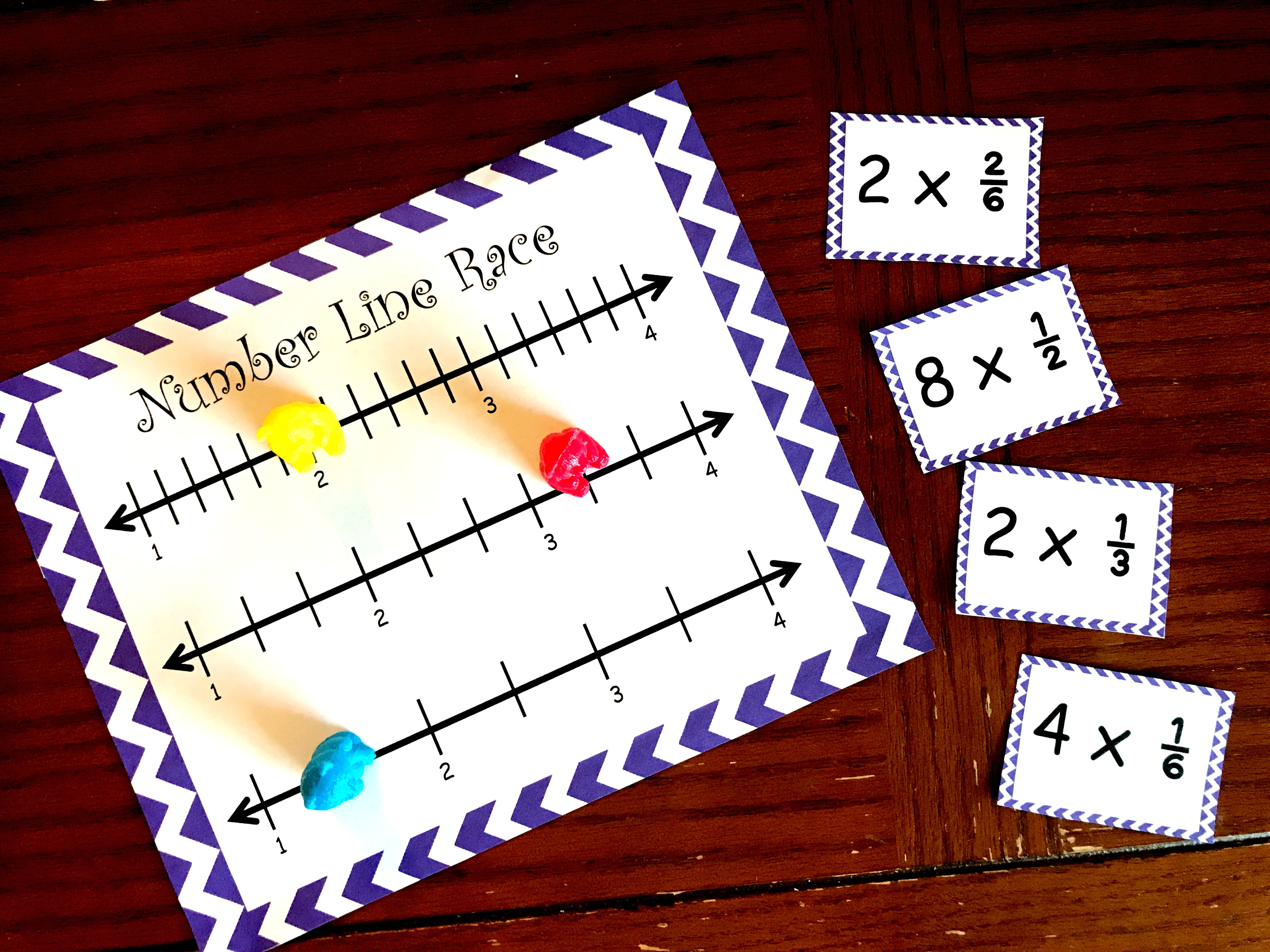Or this one that helps children understand the area models used in multiplying mixed numbers.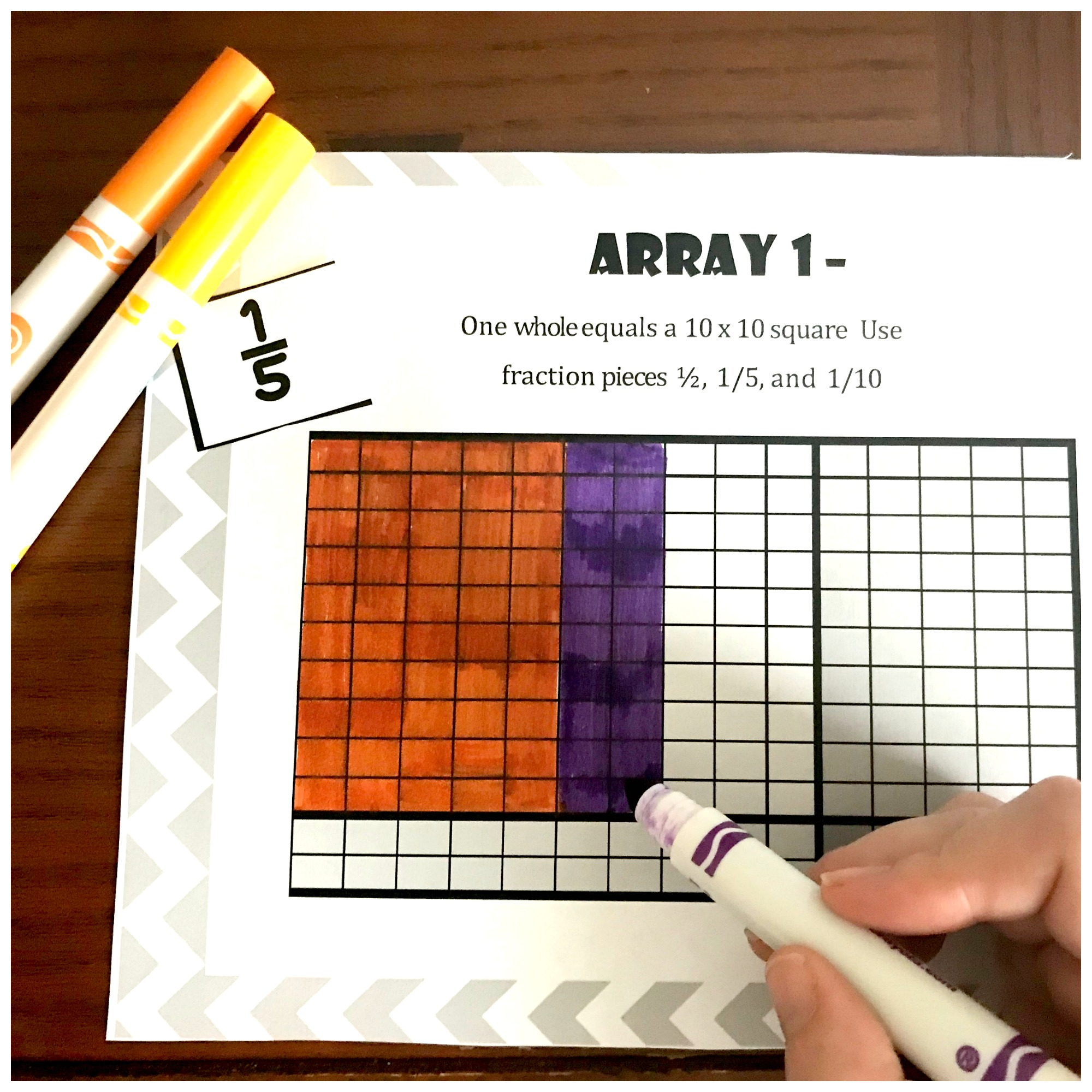Prep – Work

• Print off game boards and game cards on card stock paper… I highly recommend using a color printer for the arrays so the children can see where the fractions “overlap”
• Cut out the game cards
• Gather up game markers

Before you Play:

Playing games is always fun, but direct instruction is also very important. Before playing this game, the children need to understand two concepts.

1. First, they need to know how to multiply fractions by fractions using an algorithm.
2. They also need to be able to multiply fractions by fractions using area models

Once your little ones have learned this they are ready to practice their new skills with this game.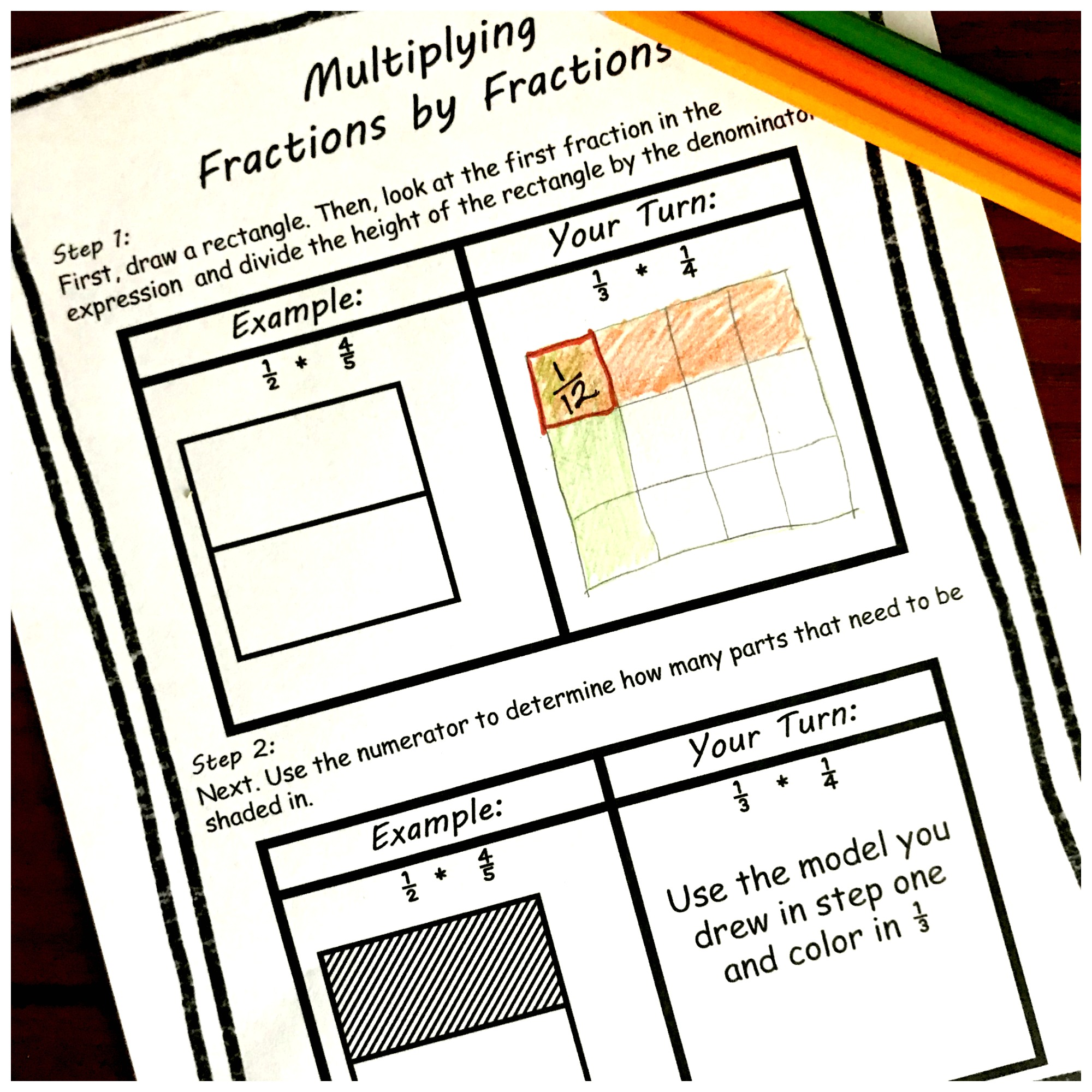How to Play Multiplying Fractions Game

1. First, draw a game card and have the children figure out the answer.

In this example, the card 1/2 x 2/3 was drawn. The children would need to solve the problem by multiplying the numerators and then the denominators to get the answer 2/6. With this game, the answers are not simplified….helping children focus on just finding the right answer. If you want them to practice this, you could always encourage them to simplify the answer verbally.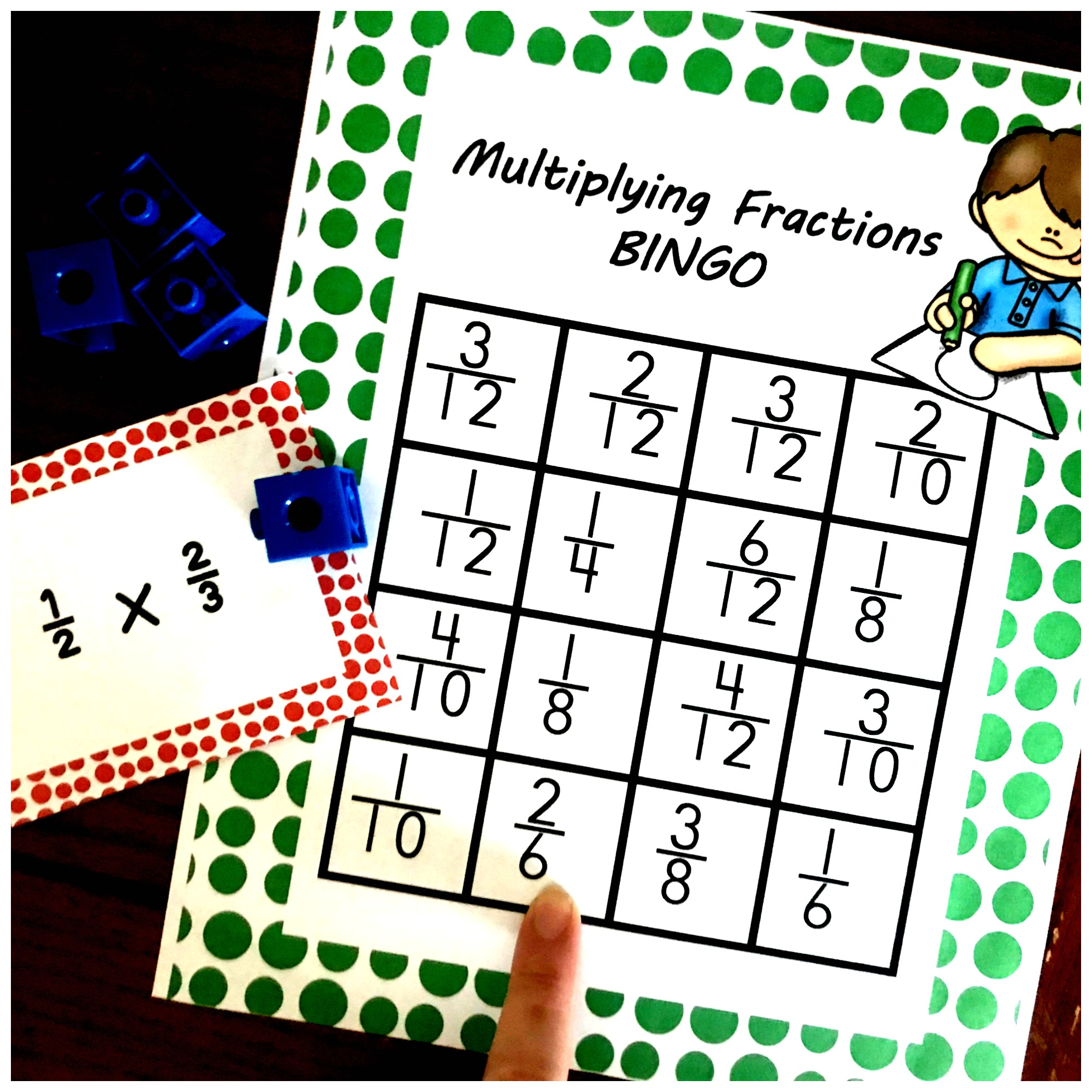This example shows an area model card being drawn. The children would need to look at the card and figure out that 1/2 is colored in and 1/3 is colored in. Then knowing that red and yellow make orange, they can see that 1 out of 6 squares have been shaded in twice. Therefore, 1/2 x 1/3 = 1/6.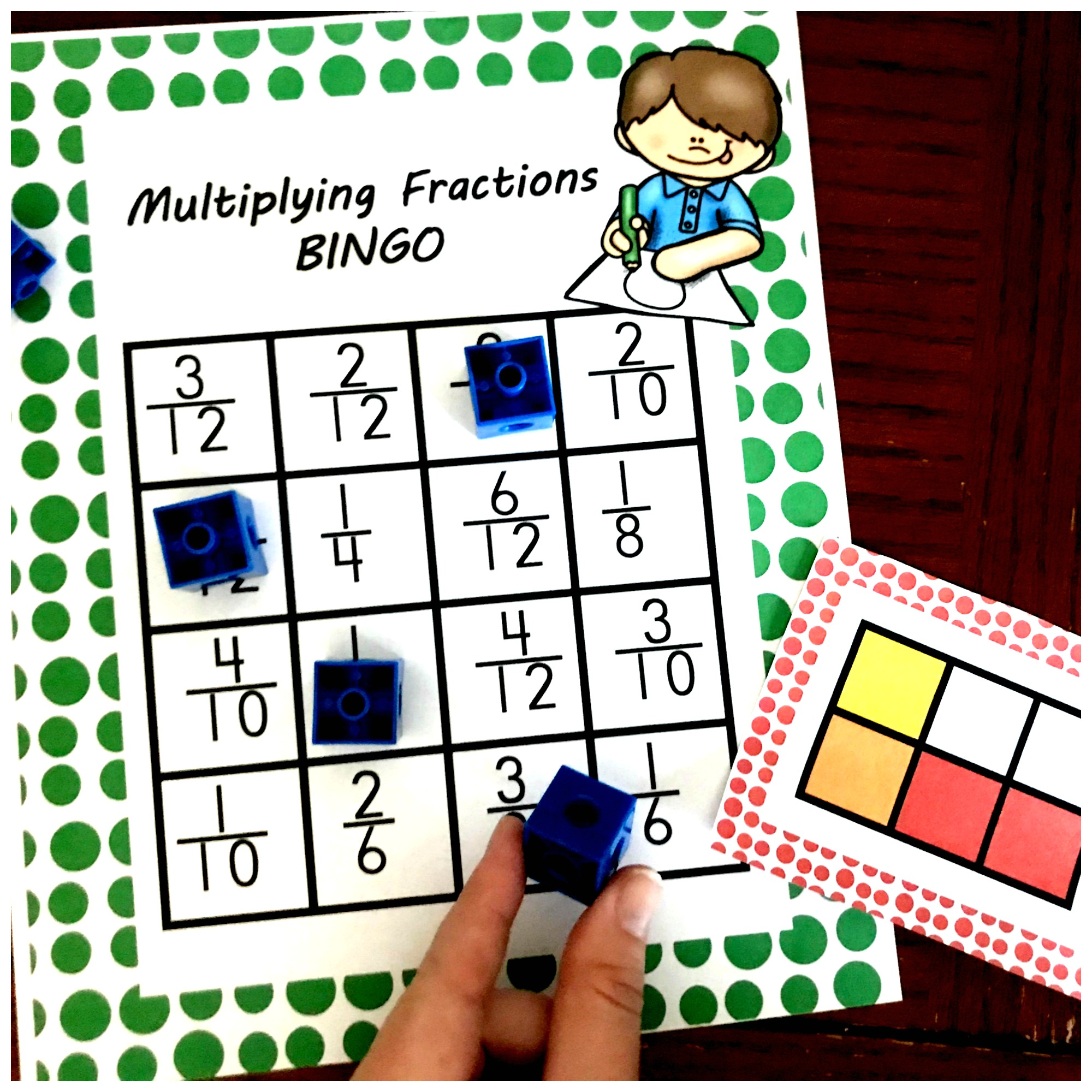2. Once the student has figured out the answer, they can find it and cover it on the game board.

3. The first child that gets five in a row wins!!

Another Option:

If you want to help your children focus on the expressions that equal a model, there is a specific board for that. You can use the same models that you used in the first game, but these boards don’t have the answers just the expression.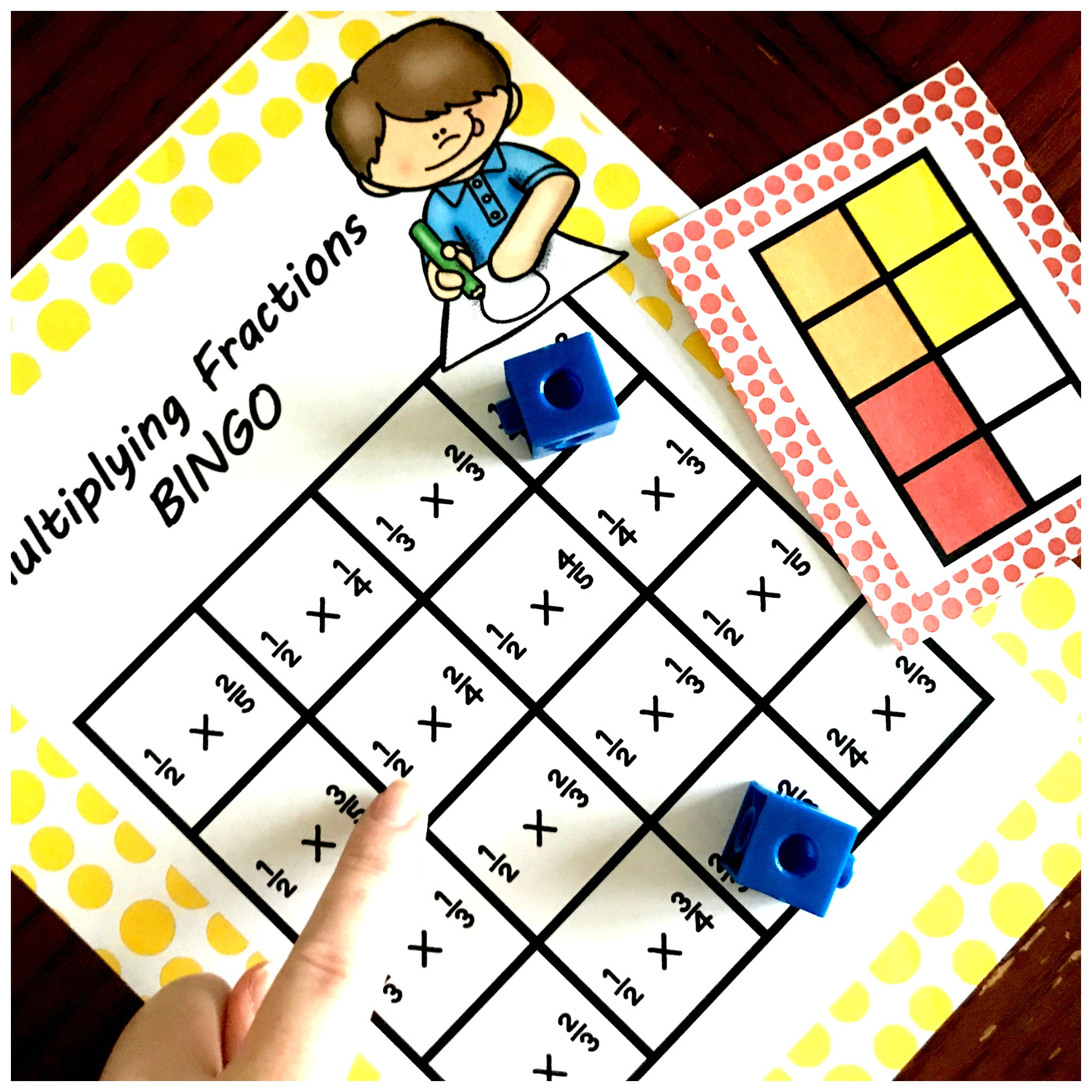Here, a model was drawn that has 1/2 colored in and 2/4 colored in. The student then needs to find the expression that says 1/2 x 2/4.

Get both games here!

So toss out all those paper and pencil drills and kill sheets, and grab some game markers for some fun practice.

You’ve Got This

Rachel